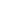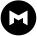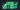# How to Avoid Inflated A/B Test ResultsJulia Glick, Senior Data Scientist

March 19, 2021 4 minute readThis is a excerpt from our latest whitepaper on A/B testing.

When we run a traditional linear regression analysis or a t-test, as we do for most A/B tests, we assume that the estimate of the difference between the treatment and control groups will be equal to the true difference in expectation.

Of course, when we actually run and analyze the test, the estimate will be off by some amount (on average, by one standard error); half the time it will be too low, and half the time it will be too high. But this all averages out in the long term, right?

Well, not always.

For most companies, the process for analyzing A/B tests is based on “null hypothesis significance testing” (NHST). But this process often comes up short.

(We’re going to have to get precise with our language in order to find the problems, so there’s some statistical jargon here, including formal definitions of things many of us are used to thinking about informally. If you have experience with A/B tests in practice, then you probably understand the concepts just fine, whether or not you’re fluent with the technical terms.)

## Taking a closer look at A/B testing based on NHST

In order to analyze a test, we construct an estimator using the observed data. Typically we’ll use a t-test or linear regression, which are both versions of “ordinary least squares” (OLS). We also estimate the uncertainty around that estimator. Together, these give us a test statistic, such as a t or F statistic, which has a known distribution if the test effect was 0, that is, under the “null hypothesis” of no difference. If the observed test statistic falls into an extreme, low-probability region under the null distribution, then we reject the null hypothesis and declare that there was a difference between the test and control groups. If the statistic is in a high-probability region of the null distribution, then we fail to reject the null hypothesis.

The power of a test to reject the null hypothesis is the probability (in advance of running the test) that the test statistic will reject, given the true difference between the groups. For a test with no difference between the groups, that probability will be equal to the probability of a “false positive” result, called 𝛼.

Test power depends on both the true and unknown difference between groups and on the uncertainty around the estimator. Since uncertainty decreases as the number of users in the test increases, we need to be sure to run tests that are large enough to detect results of business interest. But running excessively large tests incurs business costs, including opportunity costs (failing to run other tests that we could be running alongside this one) and risks (assigning too many users to a test condition that turns out to be harmful). Test power is very important, and we’re going to come back to it several times in this paper; unfortunately, it’s also a very tricky and challenging topic in practice.

No matter what, the estimate of the effect size that we get from OLS is the Best Linear Unbiased Estimator for the difference between the test and control groups. That’s true whether or not the test statistic rejects the null hypothesis.

In most business contexts, the process of analyzing a test is not used exactly as the assumptions of NHST dictate because we have many business questions that need answering. Two of those questions are usually, “Is this effect ‘real’?” and “If it’s real, how big is this effect?”

## Accounting for the statistical significance filter

Unfortunately, if we answer those questions in a naive way, we end up with what Andrew Gelman calls the “statistical significance filter”. It goes like this:

First, we ask the “is it real?” question, and we use NHST to answer the question: the effect is “real” if p<.05 (or p<𝛼 for whatever our 𝛼 is). If the effect is not “real,” then we decide it’s not worth bothering about; the effect might as well be 0 for our decisions.

If the effect is “real,” i.e., statistically significant, then we use the estimate of the effect size from the test to guide our decisions about whether to go forward with any new change, such as rolling out the test condition to all users.

The problem is that now we’re not looking at an unbiased estimator anymore. We’re not using the effect size estimate itself, we’re using the effect size estimate conditional on the test being statistically significant, and that is a totally different situation.

What happens as a result? Our effect size estimates become inflated away from 0 and we get results that are, on average, too extreme. And tests that are less powerful, but that come out significant anyway, give even more inflated results. This can make us overly optimistic about the future impact of a change, or, worse, keep us chasing product changes that do almost nothing at all instead of switching to more fruitful avenues of exploration.

If we understand and account for the statistical significance filter, however, we can avoid being led astray. Let’s walk through the problem in detail.